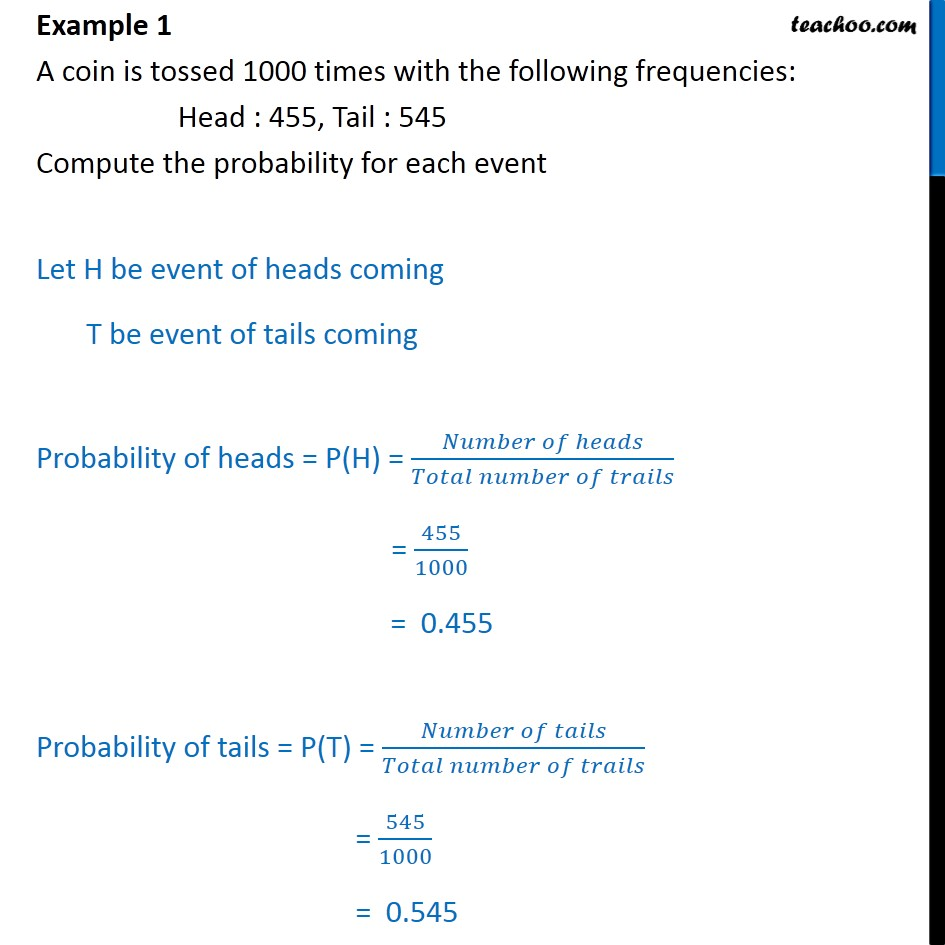1. Chapter 15 Class 9 Probability
2. Concept wise
3. Finding probability

Transcript

Example 1 A coin is tossed 1000 times with the following frequencies: Head : 455, Tail : 545 Compute the probability for each event Let H be event of heads coming T be event of tails coming Probability of heads = P(H) = ( )/( ) = 455/1000 = 0.455 Probability of tails = P(T) = ( )/( ) = 545/1000 = 0.545

Finding probability

About the AuthorDavneet Singh
Davneet Singh is a graduate from Indian Institute of Technology, Kanpur. He has been teaching from the past 10 years. He provides courses for Maths and Science at Teachoo.